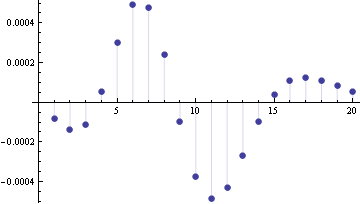# The Wilson-Hilferty approximation to the Poisson distribution

If X ~ Poisson(λ) with λ “large” then X is well approximated by a normal distribution. The approximation that falls out of the central limit theorem approximates the CDF of X by

FX(k) ≈ Φ((k + 0.5 – λ)/√ λ).

Here Φ is the CDF of a standard normal (Gaussian) random variable. The central limit theorem approximation is studied in these notes. The Wilson-Hilferty approximation improves on the classical approximation by using a non-linear transformation of the argument k. This approximation uses

FX(k) ≈ Φ((c – μ)/σ)

where

c = (λ/(1+k))1/3, μ = 1 – 1/(9k + 9), and σ = 1/(3 √(1 + k)).

## Example

The graph below gives the error for the normal approximation to the CDF of a Poission(10) random variable using FX(k) ≈ Φ((k + 0.5 – λ)/√ λ).Here is the corresponding graph using the Wilson-Hilferty approximation.The maximum error in the classical approximation is 0.0207. The maximum error in the W-H approximation is 0.00049, about 42 times smaller. This is typical: the error is often a couple orders of magnitude smaller in the W-H approximation than the classical approximation.

For more information, see “Some Suggestions for Teaching About Normal Approximation to Poisson and Binomial Distribution Functions” by Scott M. Lesch and Daniel R. Jeske, The American Statistician, August 2009, Vol 63, No 3.

See also notes on the normal approximation to the beta, binomial, gamma, and student-t distributions.